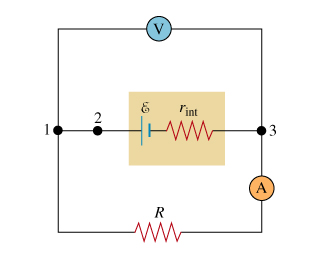# Problem: Learning Goal: To understand how to compute power dissipation in a resistive circuit.(Figure 1) The circuit in the diagram consists of a battery with EMF E, a resistor with resistance R, an ammeter, and a voltmeter. The voltmeter and the ammeter (labeled V and A) can be considered ideal; that is, their resistances are infinity and zero, respectively. The current in the resistor is l, and the voltage across it is V. The internal resistance of the battery r int is not zero.Part AWhat is the ammeter reading l?Express your answer in terms of E, R, and r int.Part BWhat is the voltmeter reading V?Express your answer in terms of E, R, and r int.Part CWhat is the power PR dissipated in the resistor?Express your answer in terms of I and VPart DAgain, what is the power PR dissipated in the resistor. This time, express your answer in terms of one or more of the following variables: l, r int, and R.Part EFor the third time, what is the power PR dissipated in the resistor?Express your answer in terms of one or more of the following variables: E, r int and R.Part FWhat is the total power P total dissipated in the resistive elements of the circuit?Express your answer in terms of one or more of the following variables: E, r int, and R.Part GWhat is the total power P total dissipated in the resistive elements of the circuit, in terms of the EMF E of the battery and the current in the circuit?Express your answer in terms of E and the ammeter current I.

###### FREE Expert Solution

Kirchhoff's voltage law:

$\overline{){\mathbf{\Sigma }}{\mathbf{V}}{\mathbf{=}}{\mathbf{0}}}$

Voltage, V = iR

Part A

Applying Kirchhoff's loop rule in the lower loop in a counterclockwise direction:

$\begin{array}{rcl}\mathbf{IR}\mathbf{+}\mathbit{I}{\mathbf{r}}_{\mathbf{i}\mathbf{n}\mathbf{t}}\mathbf{-}\mathbf{E}& \mathbf{=}& \mathbf{0}\\ \mathbit{I}\mathbf{\left(}\mathbf{R}\mathbf{+}{\mathbf{r}}_{\mathbf{i}\mathbf{n}\mathbf{t}}\mathbf{\right)}& \mathbf{=}& \mathbf{E}\end{array}$

I = E/(R + rint)

The ammeter reading is expressed as E/(R + rint).

Part B

Voltage is given by, V = IR

90% (478 ratings)###### Problem DetailsLearning Goal: To understand how to compute power dissipation in a resistive circuit.

(Figure 1) The circuit in the diagram consists of a battery with EMF E, a resistor with resistance R, an ammeter, and a voltmeter. The voltmeter and the ammeter (labeled V and A) can be considered ideal; that is, their resistances are infinity and zero, respectively. The current in the resistor is l, and the voltage across it is V. The internal resistance of the battery r int is not zero.

Part A
What is the ammeter reading l?
Express your answer in terms of E, R, and r int.

Part B
What is the voltmeter reading V?
Express your answer in terms of E, R, and r int.

Part C
What is the power PR dissipated in the resistor?
Express your answer in terms of I and V

Part D
Again, what is the power PR dissipated in the resistor. This time, express your answer in terms of one or more of the following variables: l, r int, and R.

Part E
For the third time, what is the power PR dissipated in the resistor?
Express your answer in terms of one or more of the following variables: E, r int and R.

Part F
What is the total power P total dissipated in the resistive elements of the circuit?
Express your answer in terms of one or more of the following variables: E, r int, and R.

Part G
What is the total power P total dissipated in the resistive elements of the circuit, in terms of the EMF E of the battery and the current in the circuit?
Express your answer in terms of E and the ammeter current I.

Frequently Asked Questions

What scientific concept do you need to know in order to solve this problem?

Our tutors have indicated that to solve this problem you will need to apply the Power in Circuits concept. You can view video lessons to learn Power in Circuits. Or if you need more Power in Circuits practice, you can also practice Power in Circuits practice problems.